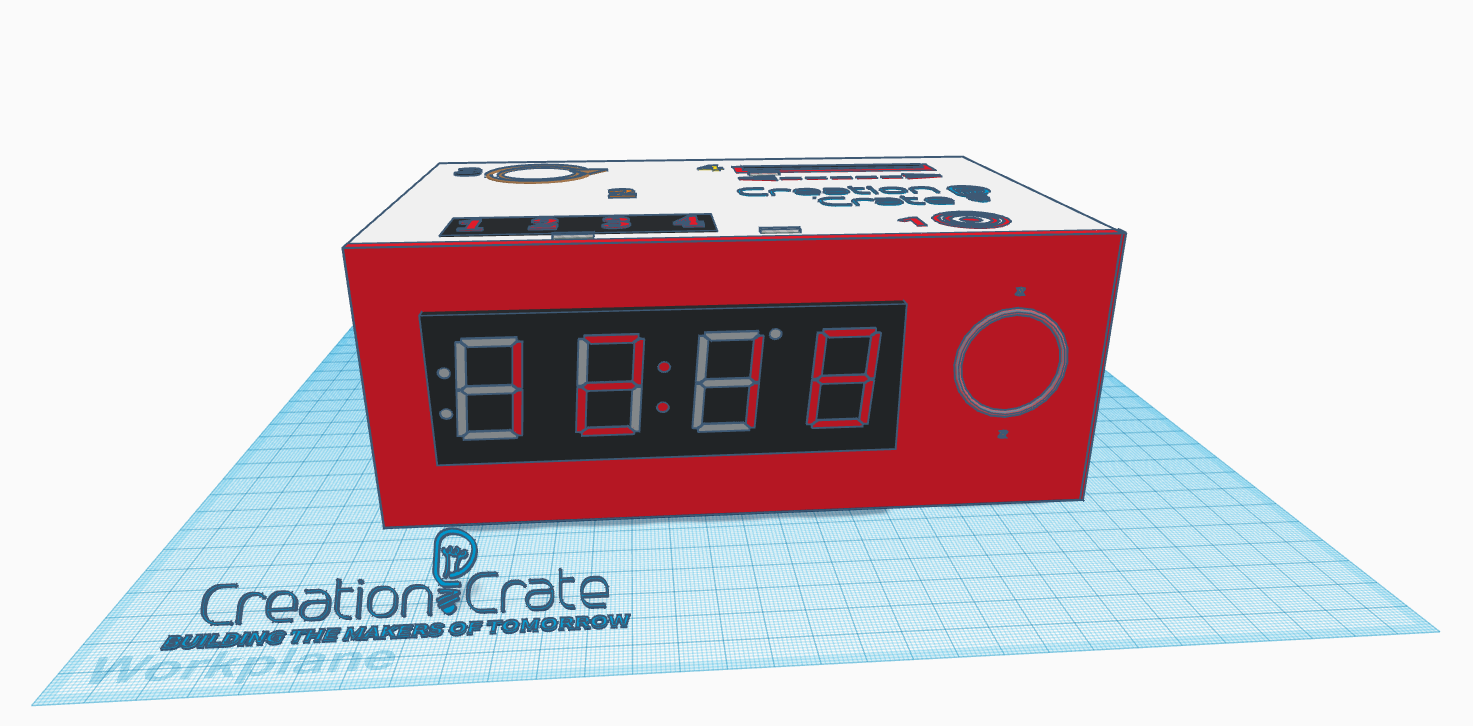# UNO MINUTO<

# EXERCISE SOLUTIONS:

Question 1

void setup()
{
Wire.begin(); //initiate the wire library for the two-wire communication
matrix.begin(0x70); //assigns an address to the I2C device, in this case the 7-segment display
DS3231_init(DS3231_INTCN); //sets up the IC on the communication bus - (what is this parameter) - initializing/ configuring I2C communication to the DS3231
memset(recv, 0, BUFF_MAX); //clear the memory - format is: memset(variable, value, sizeof(data)), here: (variable='recv', value=0, length=128 bytes)

matrix.setBrightness (05); //setting the brightness of the display here from 0-15
matrix.writeDisplay(); // sending the new value to the display

}

Question 2
/* -- DS3231 Information -- */

char recv[BUFF_MAX]; //defines variable 'recv' as an array [] of characters, 'char', with up to 128 bytes, 'BUFF_MAX' (defined in line 11)
unsigned long prev; //stores the last time we received the time from the ds3231 module
int interval = 1000; //sets the time, in milliseconds, between requests sent to the ds3231 module

int displayBright = 9 //sets the hour that the display should turn bright
int displayDim = 20 // sets the hour that the display should turn dim

THEN

}
matrix.writeDisplay();
}

if (t.hour > displayBright && t.hour < displayDim)
{ matrix.setBrightness (15); }
else
{ matrix.setBrightness(2); }
matrix.writeDisplay();
Monthly Challenge

I chose to display the different time at the top of each hour (minutes = 0) and for 10 seconds. In order to know that it was a different time, I also made the display blink. Just before the end of the loop, I checked to see if the minutes were zero. I also checked to see if the seconds were equal to zero as well, so it would not continue to call the function after the first 10 seconds. If both are true ( && ), I called a function “secondTimeZone”

if(t.min == 0 && t.sec == 00)
{
secondTimeZone(currentTime, t.hour); //Here we send the current time and hour to the function
}
} // End of the void loop()

I added this function at the end of the code:

void secondTimeZone (int cTime, int cHour)
{
int timeZone = -3; //enter the time difference in hours, +/-
int timeDiff = (timeZone*100); //puts the difference in the correct column
if ((cHour + timeDiff) > 12){ cTime -= 1200; } //Subtracts 12 from hours greater than 12
int otherTime = (cTime + timeDiff); //calculates the new time
matrix.print (otherTime);
matrix.drawColon (true); //display the colon
matrix.blinkRate (1); //blink the display so we know it's the other time zone
matrix.writeDisplay();
delay (10000); //keep the new time on display for 10 seconds